# Algebra II : Mean, Standard Deviation, and Normal Distribution

## Example Questions

### Example Question #95 : Probability

The mean of the following numbers is 13. Solve for x.

18, 15, 10, 8, x

Possible Answers:

13

15

14

12

Correct answer:

14

Explanation: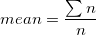where n equals the number of events.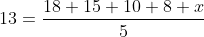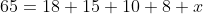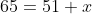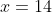### Example Question #1 : Mean, Standard Deviation, And Normal Distribution

On 3 exams, Willow has an average score of 95 points. Her lowest score was an 90. If this score is dropped, what is her new average?

Possible Answers:

98

97.5

96

100

Correct answer:

97.5

Explanation:

To answer this question, you must first calculate Willow's total points from the 3 exams. The total points will be equal to 3 times the original average:

95*3=285

Now, if the 90 is removed, his new total will be:

285-90=195

With the score dropped, her new total number of exams is 2. Therefore, the new average is: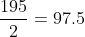### Example Question #1 : Mean, Standard Deviation, And Normal Distribution

The ages of the students at Rosa Parks High School are normally distributed with a mean of 15.5 and a standard deviation of 0.6 years.

What is the proportion of students that are older than 16.2 years old?

Possible Answers:

5%

2.5%

1%

0%

Correct answer:

2.5%

Explanation:

This question relates to the 689599.7 rule of normal distribution. We know that 95% of the data are within 2 standard deviations from the mean.

In this case this means that 95% of the students are between

15.520.6 and 15.5+20.5

151.2 and 15+1.2

13.8 and 16.2

Therefore we have 5% of the students that are outside of this range. Since the normal distribution is symmetric, the proportion of students below 13.8 is the same as the proportion of students above 16.2.

Thus the right answer is one half of 5%, or 2.5%.

2.5% of the students are older than 16.2 years old.

### Example Question #98 : Probability

The ages of the students at Central Universityl are normally distributed with a mean of 23.5 and a standard deviation of 2.5 years.

What is the proportion of students that are younger than 18.5 years old?

Possible Answers:

0%

5%

1%

2.5%

Correct answer:

2.5%

Explanation:

This question relates to the 689599.7 rule of normal distribution. We know that 95% of the data are within 2 standard deviations from the mean.

In this case this means that 95% of the students are between

23.52⋅2.5 and 23.5+2⋅2.5

23.5-5 and 23.5+5

18.5 and 28.5

Therefore we have 5% of the students that are outside of this range. Since the normal distribution is symmetric, the proportion of students below 18.5 is the same as the proportion of students above 28.5.

Thus the right answer is half of 5% or 2.5%.

### Example Question #99 : Probability

Find the standard deviation of the following set of numbers: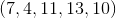Possible Answers: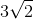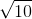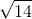Correct answer:Explanation:

To begin, we must remember the formula for standard deviation: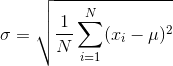where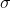is the standard deviation, N is the number of values in our set,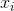is the value we're currently evaluating in the summation, and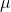is the mean of our set of numbers. All the summation part of the equation means is that we subtract our mean from each number in the set, square that value, and then add all of those values for each number together. So before we find the values that will be added together, we must first find our mean for the set of numbers: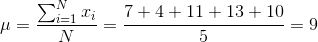Now we can determine the value for our summation for each number in the set: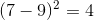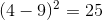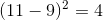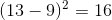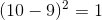Looking at our equation for standard variation, now all we must do is sum all of the values above, divide by N, and take the square root: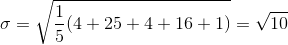### Example Question #100 : Probability

The formula for standard deviation is the following: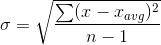.

Where,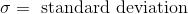,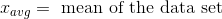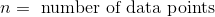Five students took a test and recieved the following grades: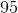,,,,. What is the standard deviation of the test grades to the nearest decimal place?

Possible Answers: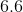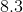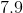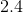None of the other answers.

Correct answer:Explanation:

The first step in solving for standrd deviation is to find the mean of the data set.

For this problem: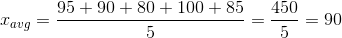.

Now we can evaluate the summation: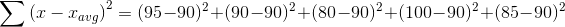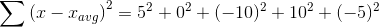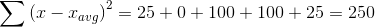Now we can rewrite the standard deviation expression: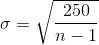There are 5 data points, so n = 5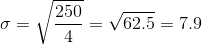### Example Question #1 : Mean, Standard Deviation, And Normal Distribution

In a normal distribution, what percentage is covered within one standard deviation?

Possible Answers:

95%

50%

99.7%

68%

34%

Correct answer:

68%

Explanation:

By drawing a bell curve, the middle line is 50%. One standard deviation left and right of the middle line is 34% each. That means one standard deviation within is 68%

### Example Question #2 : Mean, Standard Deviation, And Normal Distribution

If the mean isand the standard deviation is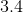, which of the following is NOT within two standard deviations?

Possible Answers: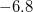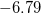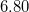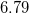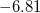Correct answer:Explanation:

Two standard deviations means to double the standard deviation value.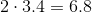This means the range isto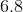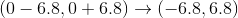Onlyis not in the range.

### Example Question #3 : Mean, Standard Deviation, And Normal Distribution

Ibram was paid $5000 for editing a 2200-page encyclopedia. What was his rate earned per page? Possible Answers:$0.56

$2.27$0.44

$1.27 Correct answer:$2.27

Explanation:

This question is really just asking for the average dollar per page. This could be easily calculated: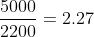However, you might also think of this as a rate problem:

D=RT

Using the information from the question, we can see that D=5000 and T=2200.

This comes out to what we solved above: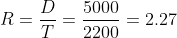### Example Question #4 : Mean, Standard Deviation, And Normal Distribution

Standard Deviation can be calculated from what statistical term?

Possible Answers:

Mode

Range

Variance

Median

Quartile

Correct answer:

Variance

Explanation:

Another way to calculate standard deviation is the square root of variance.

Variance is,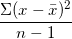.

Taking the square root of this is how standard deviation can be calculated.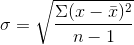### All Algebra II Resources